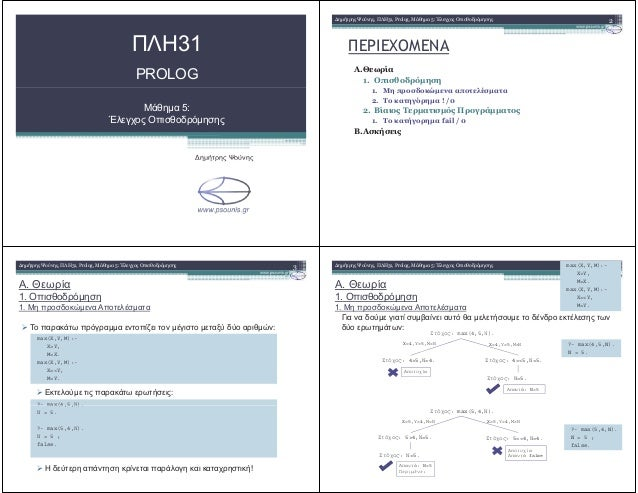Successfully reported this slideshow.
We use your LinkedIn profile and activity data to personalize ads and to show you more relevant ads. You can change your ad preferences anytime.Upcoming SlideShare
Loading in …5
×

# PROLOG ΜΑΘΗΜΑ 5 (ΕΚΤΥΠΩΣΗ)

1,999 views

Published on

A.Θεωρία
Οπισθοδρόμηση
Μη προσδοκώμενα αποτελέσματα
Το κατηγόρημα ! /0
Βίαιος Τερματισμός Προγράμματος
Το κατήγορημα fail / 0
B.Ασκήσεις

Published in: Education
• Full Name
Comment goes here.

Are you sure you want to Yes No
Your message goes here• Be the first to comment

• Be the first to like this

### PROLOG ΜΑΘΗΜΑ 5 (ΕΚΤΥΠΩΣΗ)

1. 1. 31 PROLOG 5: !"# \$%&' ( ) ' ! " # # \$ % # & '()* ! + , & # . ! 1. 1. "# \$ % " !& \$ ' # : !"# \$%&' ( ) ' (" " : max(X,Y,M):- X>Y, M=X. max(X,Y,M):- X=<Y, M=Y. ?- max(4,5, ). " ! " " " ! ?- max(4,5, ). = 5. ?- max(5,4, ). = 5 ; false. . ! 1. 1. "# \$ ) ! * + ! * * \$ " \$ : *!"# \$%&' ( ) ' : max(4,5, ). max(X,Y,M):- X>Y, M=X. max(X,Y,M):- X=<Y, M=Y. : max(4,5, ). : 4>5, =4. X=4, =5, = X=4, =5, = : 4=<5, =5. ?- max(4,5,N). N = 5. : =5. : N=5 ?- max(5,4,N). N = 5 ; false. : max(5,4, ). : 5=<4, =4. X=5, =4, = false X=5, =4, = : 5>4, =5. : =5. : N=5
2. 2. . ! 1. 2. To " ! / 0 % " ! / 0 ( + & cut) ! \$ : !"# \$%&' ( ) ' : " \$ * * . \$ , . - * ! " , * ! % !& * , * \$ * \$ ! ! * ! " . # * * " max ' :# * * " max ' : max(X,Y,M):- X>Y,!, M=X. max(X,Y,M):- X=<Y, M=Y. . ! 1. 2. To " ! / 0 . \$ * " \$ * " \$ +!"# \$%&' ( ) ' : max(4,5, ). max(X,Y,M):- X>Y,!, M=X. max(X,Y,M):- X=<Y, M=Y. : 4>5,!, =4. X=4, =5, = X=4, =5, = : 4=<5, =5. ?- max(4,5,N). N = 5. : =5. : N=5 : max(5,4, ). X=5, =4, =X=5, =4, = ?- max(5,4,N). N = 5.: 5=<4, =4. X=5, =4, =X=5, =4, = : 5>4,!, =5. : =5. : N=5 : !, =5. . ! 1. 2. To " ! / 0 * ! \$ * " /\$ . ,!"# \$%&' ( ) ' . 0 * ! # * " if-else 1 * ! * " : max(X,Y,M):- X>Y,!, M=X. max(X,Y,M):- max(X,Y,M):- X>Y,!, M=X. max(X,Y,M):- max(X,Y, ):- X>Y,!. max(X,Y, ). max(X,Y,M):- X=<Y, M=Y. max(X,Y,M):- M=Y. max(X,Y, ). . ! 2. . ! % 1. To " fail / 0 % " fail / 0 ! " * ! : -!"# \$%&' ( ) ' " \$ * * . ( "" ) * " " . 2 \$ * " * " # ! fail !& * * ! * . (! ! & " + ! * \$ ! * " \$ * " .
3. 3. .. " (, 1 " Prolog: .!"# \$%&' ( ) ' first:- room(X), write(X), nl, fail. 0 ! " : (a1) first. (a2) second.fail. second:- room(X), !, write(X), nl, fail. third:- room(X), write(X), nl, !, fail. fourth:- !, room(X), write(X), (a2) second. (a3) third. (a4) fourth. (a5) fifth. ) " \$ ! ! " : (1) (2) (3) (4) (5) a a b a a b a b write(X), nl, fail. fifth:- room(X), write(X), nl, fail, !. room(a). room(b). room(c). room(d). ) / , & * , . . a3->2 No c d No No c d No c d No .. " (, 1 /!"# \$%&' ( ) ' !"# \$%&' ( ) ' .. " (, 2 ( ) f(X,Y):- X<0,!, Y=0. ( ) f(X,Y):- X<0,!, Y=0. f(_,0). ( ) f(X,Y):- X>=0, !, Y=0. f(_,1). ( ) f(X,Y):- X<0, !, Y=0. f(_,1). ( ) f(_,1). f(X,Y):- X<0, !, Y=0. .. " (, 2 !"# \$%&' ( ) '